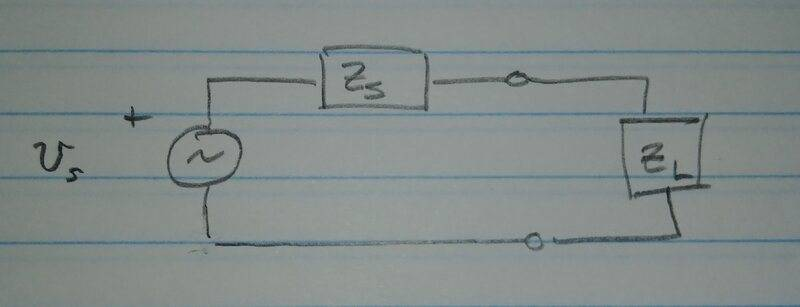# Resonance in transmission lines

ergospherical
I've calculated the relationships between the input impedance, line impedance and terminal impedance for a mismatched transmission line (parallel-wire type and of length ##l##). I'm now asked to consider the case where "the source [an oscillator] is in resonance with the line". What exactly does this condition mean (in terms of equations, preferably)?

I'm now asked to consider the case where "the source [an oscillator] is in resonance with the line".
There are a couple of possibilities with the question.

A fixed frequency oscillator could drive a line near the line's resonant frequency, so the Q of the line would then be important, and there will be multiple harmonics of line length.

Or, the combined oscillator and line could resonate together at some other frequency.
https://en.wikipedia.org/wiki/Lecher_line

••DaveE and ergospherical
Gold Member
So in either of the two scenarios @Baluncore suggested, you'll want to look at the relationship between the source impedance and the load impedance. As in the model below. In the first case ZS must be negligible w.r.t. ZL (or you have to account for it somehow). In the second case the implication is that the resonance is for the combination.

If they haven't told you what the source impedance is, I would assume the first case, otherwise it could be anything.PS: Also note that you could have more than one resonance if the total network is complicated.

•ergospherical
Gold Member
At resonance the line presents a purely resistive load to the oscillator.
If however, the oscillator is not isolated from the line by a buffer amplifier or such like, then the two resonant circuits will be coupled together. In such a case, the impedances (or admittances, depending on the circuit) of the two circuits will be added together and in general the frequency of the oscillator will change. In practice, whichever circuit has the higher Q will largely determine the oscillator freqeucncy.

•ergospherical
Gold Member
If however, the oscillator is not isolated from the line by a buffer amplifier or such like, then the two resonant circuits will be coupled together.
Yes. This is the key point and requires an analysis of the source impedance vs. the downstream stuff.

In practice, whichever circuit has the higher Q will largely determine the oscillator freqeucncy.
Yes, if there are two (or more) separate networks, or resonances. But this refers to the largest response. A lower frequency resonance can be excited by higher frequencies and will also up on the spectrum analyzer. Higher frequency resonances can also show up, excited by noise or transients.

ergospherical
At resonance the line presents a purely resistive load to the oscillator.
Thanks, yes this is how I finally arrived at the solution. It's given that an open-ended, quarter-wavelength line of length ##2a = 0.25 \ \mathrm{m}## is at resonance. When a capacitance ##C## is then connected across the end of the line, resonance occurs when the line length has been reduced to ##a##. I used the results from the previous analysis to write down\begin{align*}
\dfrac{Z_I}{Z} &= \dfrac{Z_0 \cos{ka} + iZ\sin{ka}}{Z \cos{ka} + iZ_0 \sin{ka}} \\ \\

&= \dfrac{i (Z^2 - Z_0^2) \sin{ka} \cos{ka} + Z_0 Z }{Z^2 \cos^2{ka} + Z_0^2 \sin^2{ka}}
\end{align*}where ##Z_0## is the terminal impedance, ##Z## the (real) line impedance and ##Z_I## the input impedance. The initial quarter-wavelength line condition implies that ##k = 2\pi \ \mathrm{m}^{-1}##, which means ##ka = \pi/4## and ##\sin{ka} \cos{ka} = 1/2##. Given that ##Z_0 = 1/i\omega C##, then ##Z_I## is real if\begin{align*}
i (Z^2 - Z_0^2) \sin{ka} \cos{ka} + Z_0 Z = \dfrac{i}{2} \left(Z^2 + \dfrac{1}{\omega^2 C^2} \right) - \dfrac{iZ}{\omega C}
\end{align*}is real, which is only the case if it is zero. It remains to solve\begin{align*}
Z^2 - \dfrac{2}{\omega C} Z + \dfrac{1}{\omega^2 C^2} = 0
\end{align*}Since ##\omega = ck = 2\pi c##, this becomes
\begin{align*}
Z^2 - \dfrac{1}{\pi c C} Z + \dfrac{1}{4\pi^2 c^2 C^2} = 0
\end{align*}Inserting the value for ##C## gives, to my surprise, the correct answer.•DaveE and hutchphd